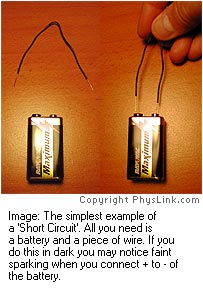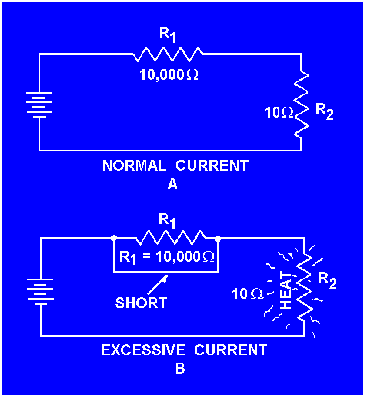# what is short circuit ?

When current in a circuit takes unintended path we call it short circuiting. For example, in a torch circuit if somehow the two terminals of the bulb touch each other the bulb will not glow. Also if somehow the two terminals of the battery touch each other the torch won't work. This condition is called short circuiting.

• 22

pls. urgent

• -9

A short circuit is simply a low resistance connection between the two conductors supplying electrical power to any circuit. This results in excessive current flow in the power source through the 'short,' and may even cause the power source to be destroyed. If a fuse is in the supply circuit, it will do its job and blow out, opening the circuit and stopping the current flow.• 4

An electric circuit is the path that an electric current takes. The current flows from one side of the source through a conductor such as wire through a resistance, such as an appliance, to the other side of the source. A short circuit occurs when the current find a path without resistance. Then large amounts of current flow and can burn insulation and start a fire.

• 1

A short circuit is an accidental path of low resistance which passes an abnormally high amount of current. A short circuit exists whenever the resistance of a circuit or the resistance of a part of a circuit drops in value to almost zero ohms. A short circuit often occurs as a result of improper wiring or broken insulation.• 2

A short circuit is an abnormal connection between two nodes of an electric circuit intended to be at different voltages. This results in an excessive electric current/overcurrent limited only by the Thévenin equivalent resistance of the rest of the network and potentially causes circuit damage, overheating, fire or explosion. Although usually the result of a fault, there are cases where short circuits are caused intentionally, for example, for the purpose of voltage-sensing crowbar circuit protectors. In the term short circuit is used by analogy to designate a connection between two nodes. This forces the two nodes to be at the same voltage. In an ideal short circuit, this means there is no resistance and no voltage drop across the short. In simple circuit analysis, wires are considered to be shorts. In real circuits, the result is a connection of nearly zero and almost no resistance. In such a case, the current drawn is limited by the rest of the circuit.

• -6
What are you looking for?